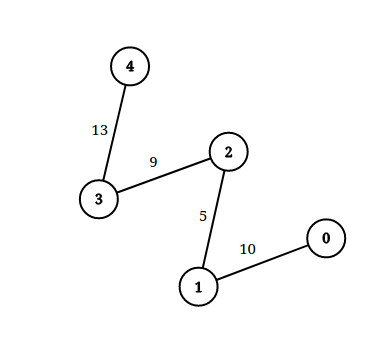Formatted question description: https://leetcode.ca/all/1697.html

# 1697. Checking Existence of Edge Length Limited Paths (Hard)

An undirected graph of n nodes is defined by edgeList, where edgeList[i] = [ui, vi, disi] denotes an edge between nodes ui and vi with distance disi. Note that there may be multiple edges between two nodes.

Given an array queries, where queries[j] = [pj, qj, limitj], your task is to determine for each queries[j] whether there is a path between pj and qj such that each edge on the path has a distance strictly less than limitj .

Return a boolean array answer, where answer.length == queries.length and the jth value of answer is true if there is a path for queries[j] is true, and false otherwise.

Example 1:Input: n = 3, edgeList = [[0,1,2],[1,2,4],[2,0,8],[1,0,16]], queries = [[0,1,2],[0,2,5]]
Output: [false,true]
Explanation: The above figure shows the given graph. Note that there are two overlapping edges between 0 and 1 with distances 2 and 16.
For the first query, between 0 and 1 there is no path where each distance is less than 2, thus we return false for this query.
For the second query, there is a path (0 -> 1 -> 2) of two edges with distances less than 5, thus we return true for this query.


Example 2:Input: n = 5, edgeList = [[0,1,10],[1,2,5],[2,3,9],[3,4,13]], queries = [[0,4,14],[1,4,13]]
Output: [true,false]
Exaplanation: The above figure shows the given graph.


Constraints:

• 2 <= n <= 105
• 1 <= edgeList.length, queries.length <= 105
• edgeList[i].length == 3
• queries[j].length == 3
• 0 <= ui, vi, pj, qj <= n - 1
• ui != vi
• pj != qj
• 1 <= disi, limitj <= 109
• There may be multiple edges between two nodes.

Related Topics:
Sort, Union Find

## Solution 1. Union Find

Sort the edges from small distance to large distance.

Traverse the queries from small limit to large limit. Given a limit, we union the nodes that can be connected.

In this way, we just need to traverse the edges that are smaller than the limit. Since we are visiting the queries with increasing limit, we just need to visit the edges once from small distance to large distance.

// OJ: https://leetcode.com/problems/checking-existence-of-edge-length-limited-paths/

// Time: O(ElogE + QlogQ)
// Space: O(N)
class UnionFind {
vector<int> id;
public:
UnionFind(int n) : id(n) {
iota(begin(id), end(id), 0);
}
void connect(int a, int b) {
int x = find(a), y = find(b);
if (x == y) return;
id[x] = y;
}
bool connected(int i, int j) { return find(i) == find(j); }
int find(int a) {
return id[a] == a ? a : (id[a] = find(id[a]));
}
};
class Solution {
public:
vector<bool> distanceLimitedPathsExist(int n, vector<vector<int>>& E, vector<vector<int>>& Q) {
vector<bool> ans(Q.size());
for (int i = 0; i < Q.size(); ++i) Q[i].push_back(i);
sort(begin(Q), end(Q), [&](auto &a, auto &b) { return a < b; });
sort(begin(E), end(E), [&](auto &a, auto &b) { return a < b; });
UnionFind uf(n);
int i = 0;
for (auto &q : Q) { // traverse the queries from small limit to large limit
int u = q, v = q, limit = q, qid = q;
for (; i < E.size() && E[i] < limit; ++i) uf.connect(E[i], E[i]); // visit the edges that are smaller than the limit
ans[qid] = uf.connected(u, v);
}
return ans;
}
};


Java

class Solution {
public boolean[] distanceLimitedPathsExist(int n, int[][] edgeList, int[][] queries) {
int queriesCount = queries.length;
Integer[] queryIndices = new Integer[queriesCount];
for (int i = 0; i < queriesCount; i++)
queryIndices[i] = i;
Arrays.sort(queryIndices, new Comparator<Integer>() {
public int compare(Integer index1, Integer index2) {
return queries[index1] - queries[index2];
}
});
Arrays.sort(edgeList, new Comparator<int[]>() {
public int compare(int[] edge1, int[] edge2) {
return edge1 - edge2;
}
});
boolean[] exists = new boolean[queriesCount];
int[] parent = new int[n];
for (int i = 0; i < n; i++)
parent[i] = i;
int index = 0;
int edgesCount = edgeList.length;
for (int i = 0; i < queriesCount; i++) {
int queryIndex = queryIndices[i];
int[] query = queries[queryIndex];
int start = query, end = query;
while (index < edgesCount && edgeList[index] < query) {
union(parent, edgeList[index], edgeList[index]);
index++;
}
exists[queryIndex] = find(parent, start) == find(parent, end);
}
return exists;
}

public void union(int[] parent, int index1, int index2) {
parent[find(parent, index1)] = find(parent, index2);
}

public int find(int[] parent, int index) {
if (parent[index] != index)
parent[index] = find(parent, parent[index]);
return parent[index];
}
}